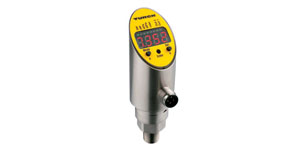What Are The Different Types Of Pressure?

TURCK 3/14/2018Read below for details on specific types and measurements of pressure.

PSI (Pounds per Square Inch): This is the unit of measure for one pound of force applied to one square inch of area. PSI is a typical unit of pressure in the United States.

BAR: One bar is equal to the atmospheric pressure on the earth at sea level. BAR was a unit of measure created in Europe and is still commonly used there.

PA (Pascal): This is the unit of measure for one newton of pressure per square meter.

InHg (Inches of Mercury): This is the unit of measure for a one inch circular column of mercury, one inch tall, at gravity and 0 °C (32 °F). InHg is typically used as a unit of measure for barometric pressure.

Torr: This unit was initially developed as a millimeter of mercury and is a very small fraction of atmospheric pressure.

InH2O (Inches of Water): This is the unit of measure for a one inch circular column of water, one inch tall, at gravity and 4 °C (39.2 °F). InH2O is typically used for a differential pressure measurement or in low pressure water applications, such as level.